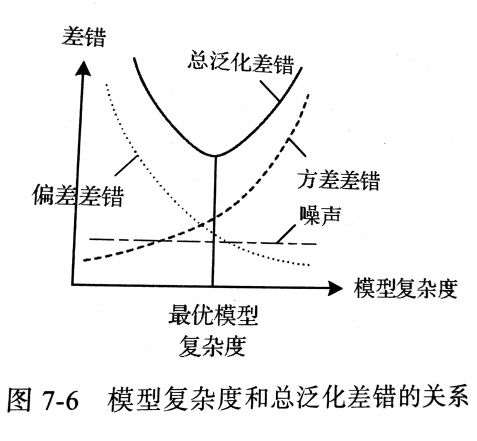全连接神经网络学习笔记

全连接神经网络前馈神经网络• 线性层和卷积层：这两种层对输入进行线性计算。层内维护着线性运算的权重
• 激活层：这层对数据进行非线性运算。非线性运算时可以逐元素非线性运算的，也可以是其它类习惯的非线性运算
• 归一化层：根据输入的均值和方差对数据进行归一化，使得数据的范围在一个相对固定的范围内
• 池化层和视觉层：这两种层和数据重采样有关，包括对数据进行下采样（就是隔几个数据采一个数据）、上采样（把一个数据复制出很多份）和重新排序。
• 丢弃层：在输入中随机选择一些输出
• 补齐层：采用循环补齐等方法让数据变多使用torch.nn.Sequential类搭建前馈神经网络torch.nn.Sequential类是由torch.nn.Module类派生得到的。torch.nn.Moudle类及其子类有以下用途。

• 表示一个神经网络 例如torch.nn.Sequential类可以表示一个前馈神经网络
• 表示神经网络的一个层 例如上次说的torch.nn.Linear就是表示神经网络的一个层的线性连接部分，它是一种线性层；torch.nn.ReLU类表示逐元素计算max(·, 0)，它是一种激活层。
• 表示损失。torch.nn.MSELoss类、torch.nn.L1Loss类、torch.nn.SmoothL1Loss类等等
from torch.nn import Linear, ReLU, Sequential

net = Sequential(Linear(3, 4), ReLU(), Linear(4, 2), ReLU())
print(net)

Sequential(
(0): Linear(in_features=3, out_features=4, bias=True)
(1): ReLU()
(2): Linear(in_features=4, out_features=2, bias=True)
(3): ReLU()
)

全连接层和全连接神经网络全连接层的意思全连接神经网络的意思非线性激活非线性激活的必要性激活层分为两大类1. 基于逐元素非线性运算的激活层。对于这类激活层，一个称为“激活函数”的非线性函数对张量进行逐元素运算。例如可以对张量求(x, max)，或是逐元素求expit()。
2. 多元素组合运算的激活层，对于这类激活层，并不是“激活函数”对张量逐元素运算，而是利用多个元素的值联合计算。例如对某些元素联合起来进行softmax()运算。

逐元素激活• S形激活
• 单侧激活
• 皱缩激活
S型激活S型激活函数把(-∞, +∞)范围的值映射到一个有限的闭区间里。从这个意义来看，S型激活可以有效控制输出的范围。但是S型激活函数常常在输入的绝对值比较大的时候导数为零，从而导致梯度消失

$$soft z = {z \over {1+|z|}}$$ (-1, 1) torch.nn.Softsign
$$expitz = {1 \over {1+exp(-z)}}$$ (0, 1) torch.nn.Sigmoid
$$tanh z = {exp(z) - exp(-z) \over {exp(z) + exp(-z)}}$$ (-1, 1) torch.nn.Hardtanh
$$hardtanh z = \begin{cases} 1, & z > 1 \\ z, & -1 \leq z \leq 1 \\ -1, & z > 6 \end{cases}$$ [-1, 1] torch.nn.Hardtanh
$$relu6 z = \begin{cases} 0, & z < 0 \\ z, & 0 \leq z \leq 6 \\ 6, & z > 6 \end{cases}$$ [0, 6] torch.nn.ReLU6

单侧激活函数Pytorch提供的单侧激活函数：

$$relu z = \begin{cases} 0, & z < 0 \\ z, & z \geq 0 \end{cases}$$ [0, +∞) torch.nn.ReLU
$$leakyrelu (z; a) = \begin{cases} az, & z < 0 \\ z, & z \geq 0 \end{cases}$$ (-∞, +∞) torch.nn.LeakyReLU
torch.nn.RReLU
torch.nn.PReLU
$$threshold(z; \lambda, \nu) = \begin{cases} \nu, & z < \lambda \\ z, & z \geq \lambda \end{cases}$$ $$\{\nu\}$$ $$\bigcup$$ [ $$\lambda, +∞$$ ) torch.nn.Threshold
$$selu (z;\sigma, \alpha) = \begin{cases} \sigma\alpha(exp(z)-1), & z < 0 \\ \sigma z, & z \geq 0 \end{cases}$$ [- $\sigma\alpha$ , +∞) torch.nn.ELU
torch.nn.SELU
$$softplus (z;\beta) = {{1 \over \beta} ln(1 + exp(\beta z))}$$ (0, +∞) torch.nn.Softplus
$ln expit(z) = -ln(1+exp(-z))$ (-∞, 0) torch.nn.LogSigmoid

• Leaky ReLU：构造类实例的时候需要传入α的值，一旦传入，不可更改（默认为0.01）
• RReLU：构造时需要传入两个参数lower和upper，α将是lower和upper间均匀分布的随机数，lower的值默认为1/8，upper的默认值为1/3
• PReLU：斜率α作为一个可优化的值，将在确定权重时一并确定。并且每个元素九可以使用不同的权重值
皱缩激活网络结构的选择欠拟合与过拟合训练集、验证集和测试集• 如果某种结构的网络的学习曲线和验证曲线都收敛到同一个比较大的差错值，通过改大网络可以使得学习曲线和验证曲线收敛到的差错值变小，那么在改大网络前就应该出现了高偏差差错，出现了欠拟合。
• 如果某种结构的网络的学习曲线和验证曲线最终值差别较大，通过改小网络可以使得这个差别变小，那么在改小网络前就应该出现了高方差差错，出现了过拟合。

例子：基于全连接网络的非线性回归数据生成和数据集分割import torch
torch.manual_seed(seed=0)# 固定随机数种子,这样生成的数据是确定的
sample_num=1000 # 生成样本数
features=torch.rand(sample_num,2)*12-6 # 特征数据
noises=torch.randn(sample_num)
def himmelblau(x):
return(x[:,0] **2 + x[:,1]-11)**2 +(x[:,0] + x[:,1] **2-7)**2
hims=himmelblau(features)*0.01
labels=hims + noises # 标签数据

train_num,validate_num,test_num=600,200,200 # 分割数据
train_mse=(noises[:train_num] **2).mean()
validate_mse=(noises[train_num:-test_num] **2).mean()
test_mse=(noises[-test_num:] **2).mean()
# MSE算法这里吧预测值当作0，平方的平均值就是数据的MSE
print('真实:训练集MSE={:g},验证集MSE={:g},测试集MSE={:g}'.format(train_mse,validate_mse,test_mse))

确定网络结构并训练网络import torch.nn as nn
# 指定隐含层数
hidden_features = [6, 2]
layers = [nn.Linear(2, hidden_features), ]

for idx, hidden_feature in enumerate(hidden_features) :
layers.append(nn.Sigmoid())
next_hidden_feature = hidden_features[idx + 1] \
if idx + 1 < len(hidden_features) else 1
layers.append(nn.Linear(hidden_feature, next_hidden_feature))
print(layers)
net = nn.Sequential(*layers)
print(f'神经网络为{format(net)}')

# 在3.5版本开始，python对星号增加新的适用场景，即在元组、列表、集合和字典内部进行对可迭代参数直接解包，
# 这里需要一再强调的是，这里是在上述四个场景下才可以对可迭代参数直接解包，
# 在其他场景下进行可迭代对象的星号解包操作时不允许的。

[Linear(in_features=2, out_features=6, bias=True), Sigmoid(), Linear(in_features=6, out_features=2, bias=True), Sigmoid(), Linear(in_features=2, out_features=1, bias=True)]

(0): Linear(in_features=2, out_features=6, bias=True)
(1): Sigmoid()
(2): Linear(in_features=6, out_features=2, bias=True)
(3): Sigmoid()
(4): Linear(in_features=2, out_features=1, bias=True)
)
import torch.optim

criterion = nn.MSELoss()

train_entry_num = 600 # 选择训练样本数

n_iter = 100000 # 最大迭代次数
for step in range(n_iter):
outputs = net(features)
# 去掉所有维度为1的维度
preds = outputs.squeeze()

loss_train = criterion(preds[:train_entry_num], labels[:train_entry_num])
loss_validate = criterion(preds[train_num: -test_num], labels[train_num: -test_num])

if step % 1000 == 0:
print('#{} 训练集MSE = {:g}，验证集MSE={:g}'.format(step, loss_train, loss_validate))

loss_train.backward()
optimizer.step()

print(f'训练集MSE = {loss_train}, 验证集MSE={loss_validate}')

#96000 训练集MSE = 1.04245，验证集MSE=1.06843
#97000 训练集MSE = 1.04209，验证集MSE=1.06864
#98000 训练集MSE = 1.04173，验证集MSE=1.06773
#99000 训练集MSE = 1.04131，验证集MSE=1.0668

outputs = net(features)
preds = outputs.squeeze()
loss = criterion(preds[-test_num:], labels[-test_num:])
print(loss)